# Alter System Examples in Oracle

## Alter System Examples in Oracle

In this article, I am going to discuss Alter System Examples in Oracle. Please read our previous article where we discussed Non-Default Name for Pfile in Oracle with Examples

##### Alter System Examples in Oracle

Until now we have learnt how to start the database using pfile. In this article, we will try to learn about alter statement examples. We have already learnt the term called scope. In the ALTER SYSTEM command, we have a clause called Scope, and this is very important and we have many options for the scope.

1. MEMORY
2. SPFILE
3. BOTH
4. DEFERRED

Memory: We can run a command with alter system and change the parameter on the memory scope. This indicates that the changes are made in memory, only take effect immediately and persist until that database is shut down. For Example, when you change a parameter and the Scope is memory, these changes happen in their memory. Only if you switch off your database and open it again, these changes will be no longer available.

SP File: This scope indicates that the change is made on the Server parameter file. The new changes will affect only when the database is next, shut down and start up again. We have some parameters. We cannot change it except in the S.P. file. So, you must change it before and then you have to restart the database again.

BOTH: We have both and this is the default. So, if you make alter system and you did not specify memory or S.B file, the default will be both. And this indicates that the change is made in memory and in the server parameter file and this is the default.

DEFERRED: We also have deferred and this means effective for future decisions. So, this means that you can make alter and you will use the option deferred, but only the future decision will take these changes.

##### MEMORY:

Now, let us try to understand scope=memory with an example.

Step1: Open the Linux machine and open the terminal. Connect to the database as “sqlplus / as sysdba”.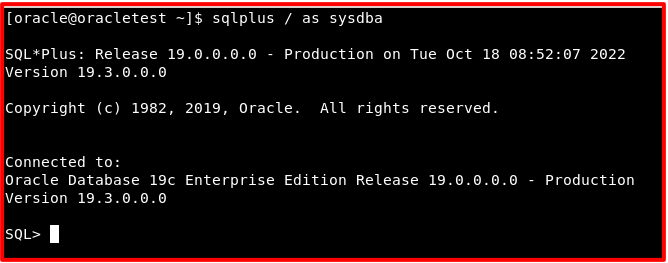Step2: Take any parameter for example max_idle_time. Let us check the value of the parameter using the command “show parameter max_idle_time”.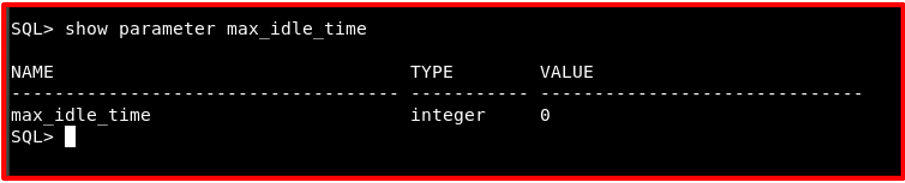We can see the value of the max_idle_time is 0. It indicates that there is no time limit for the users.

###### Step3: Alter system statement using scope = memory

So, we will try to change the value of the parameter using the alter system command.

Command: alter system set max_idle_time=20 scope=memory;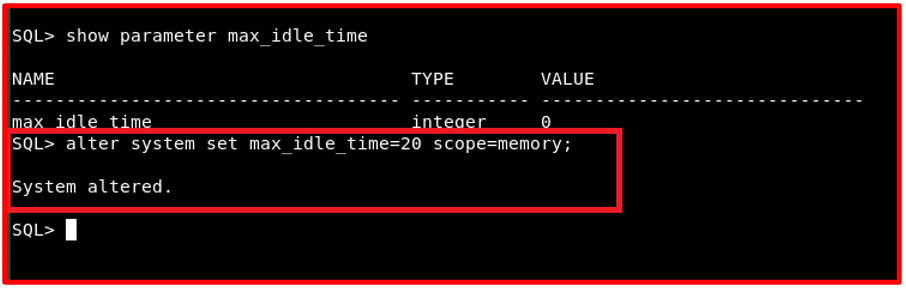So, now we have changed the value of the parameter is changed to 20 and we have mentioned scope as memory. These changes will not be written in the spfile. The changes with scope=memory will only affect the memory region. This change is only present in the instance.

###### Step4: Verify the changes in the spfile.

For reference, we can check the spfile if the change that we have made is present in the spfile or not. Go to the below-mentioned path and open the spfilejayadb.ora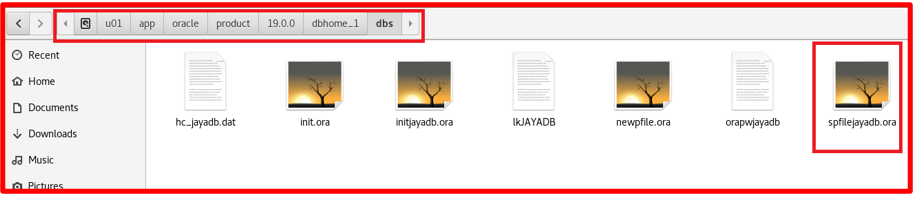Once you open the spfile you can check for the modified parameter spfile.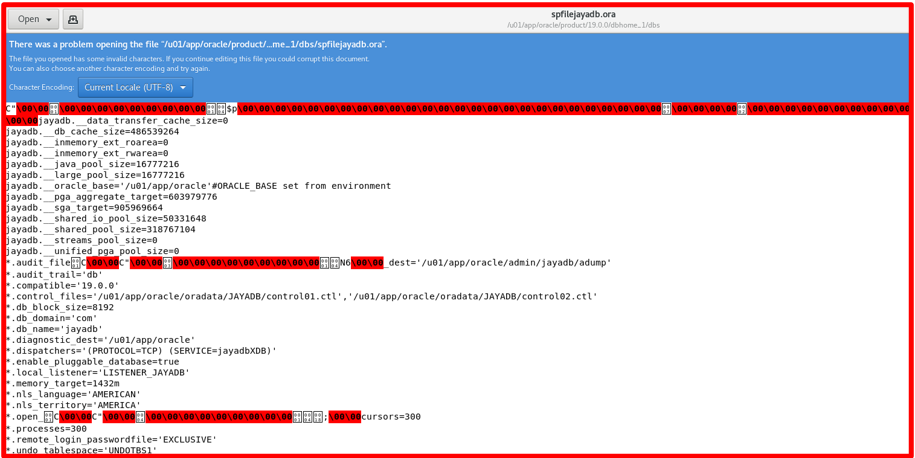We do not find the max_idle_time in the spfile. So, the changes don’t affect the spfile.

###### Step5: Verify the parameter at the database level.

Let us go ahead and check the value of the parameter max_idle_time at the database level.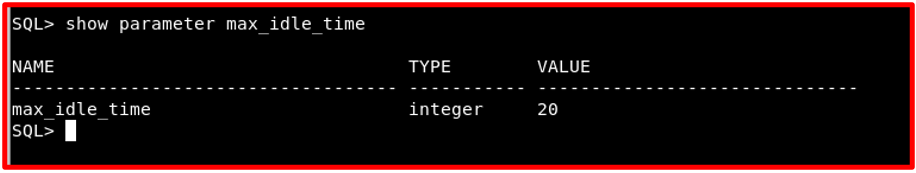We can see the max_idle_time is 20. This indicates the changes are effective only at the memory level. If we start the database again the value of the max_idle_time will get back to 0. Any new session will take the new max_idle_time value. For example, open a new terminal and connect to the database as “Sqlplus / as sysdba” and check the parameter max_idle_time.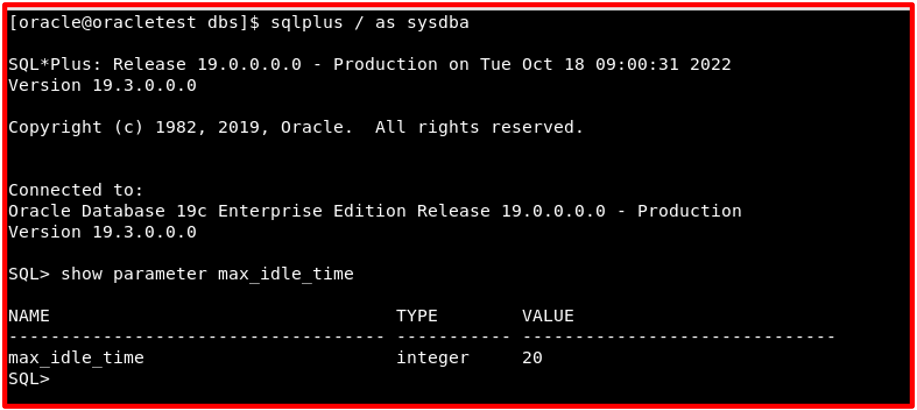Let us check the value of the parameter max_idle_time in another way using the data dictionary V\$PARAMETER and V\$SYSTEM_PARAMETER.

Command: select value from v\$parameter where name=’max_idle_time’;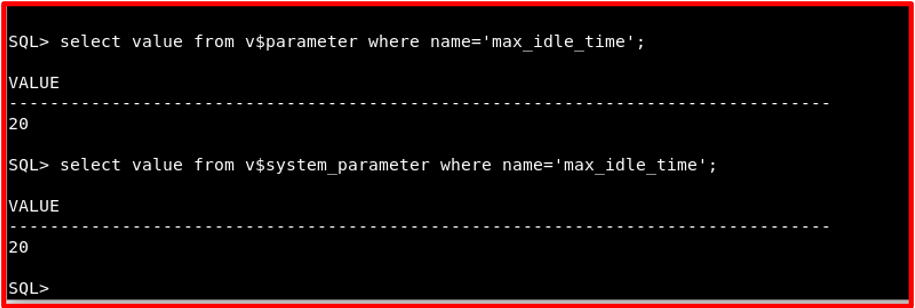From the output, we can see the value of max_idle_time is 20 for the view V\$PARAMETER and V\$SYSTEM_PARAMETER. So, any new sessions will inherit this value.

###### Step6: Restart the Database and check the parameter again.

Now let us shut down the database and start the database normally without using any pfile.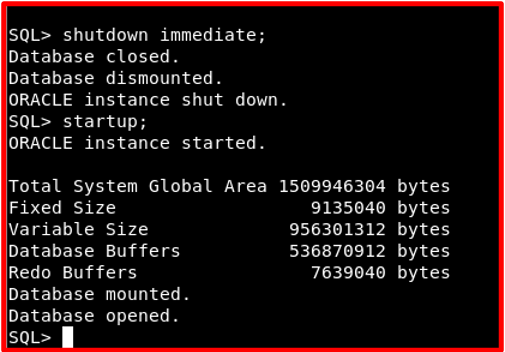We have started the database normally so the instance searches for the spfile and the spfile is present so the instance will start with spfile. We don’t have any value for max_idle_time in spfile. So, the value max_idle_time will restore back to 0 which is the instance default value. We will check the value of the parameter with the view V\$SYSTEM_PARAMETER.

Query: select value from v\$system_parameter where name=’max_idle_time’;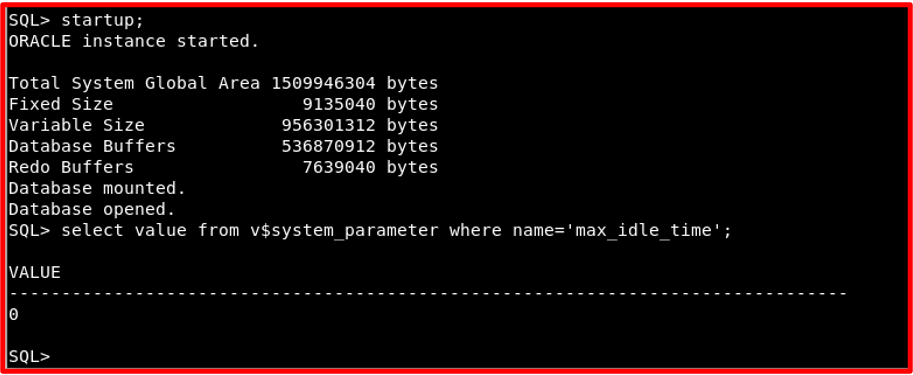We can see the value is 0 because the instance reads the spfile where we don’t have any value of the max_idle_time. Now, we will try to learn with scope =spfile.

##### SPFILE:

We will see the same alter statement with scope=spfile.

Step1: Check the existence of the parameter in the spfile. Go to the location of the spfile as shown below.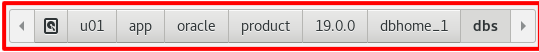Open the spfile and check for the value max_idle_time.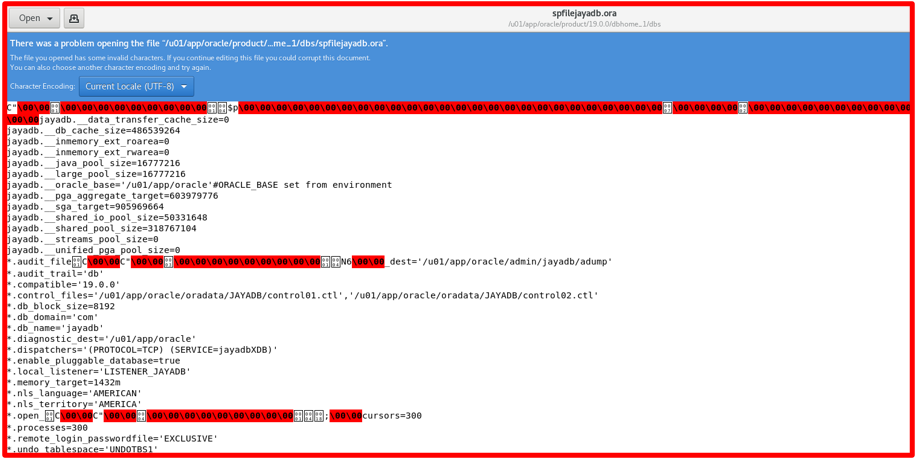###### Step2: Change the value of the parameter at the database level.

Now, open a terminal and connect to the database as “sqlplus / as sysdba”. Once you are connected to the database change the value of max_idle_time using the below command where scope=spfile.

Command: alter system set max_idle_time=50 scope=spfile;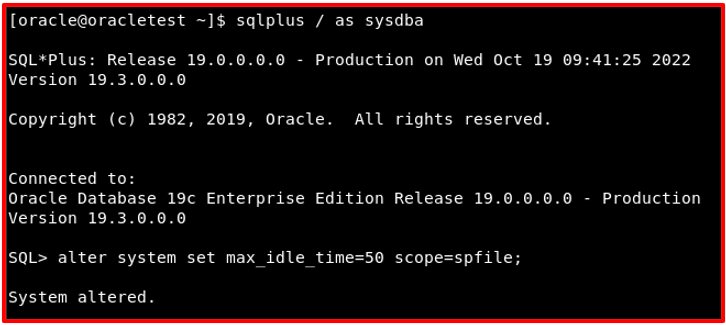This means that the changes will be reflected in spfile. But the changes will not affect immediately. The changes will affect only after the database has been restarted with spfile. Let us check whether the value of the parameter max_idle_time is changing immediately using the query.

Query: select value from v\$system_parameter where name=’max_idle_time’;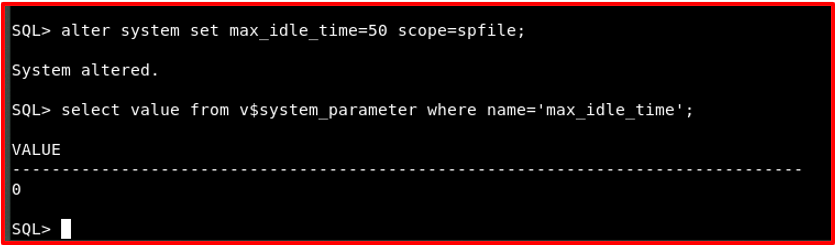Step3: Let us go ahead and check the spfile if the change that we have made has applied correctly or not.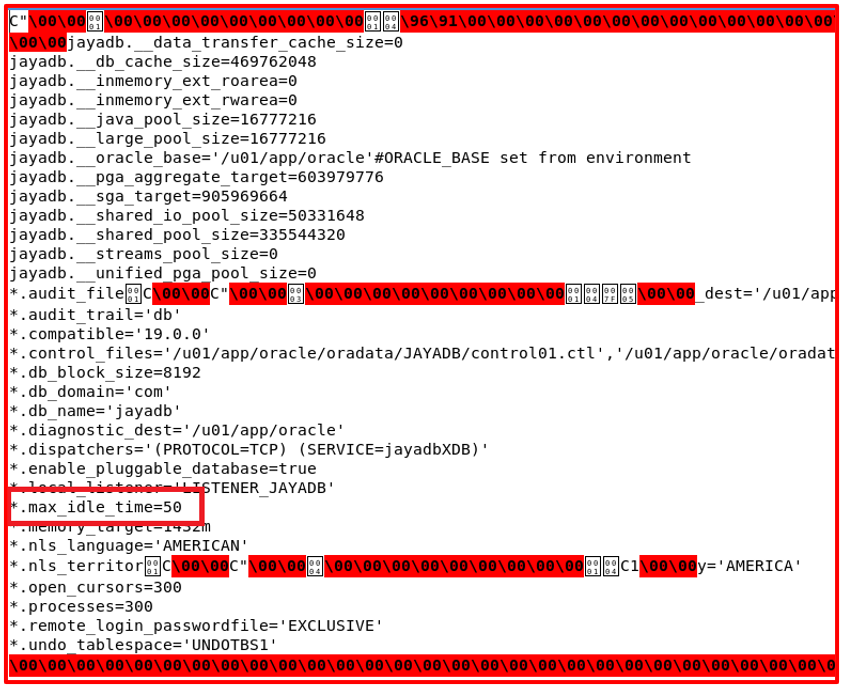We can see the value of max_idle_time= 50 in the spfile. But we do not see the same value at the database level. We must restart the database to get the value changed.

###### Step4: Restart the database and check the value of max_idle_time.

Now, let us shut down the database again and start the database normally.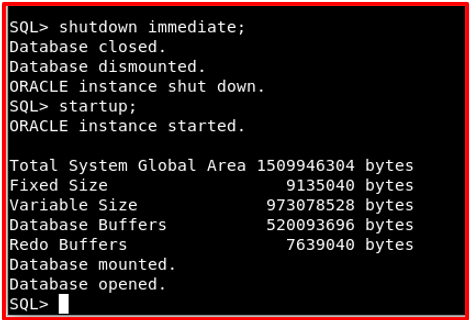Now, the database is restarted. In the background, the instance reads the spfile where we have the new value of max_idle_time. And the changes will be affected the entire database. Let us check the value of max_idle_time using the query.

Query: select value from v\$system_parameter where name=’max_idle_time’;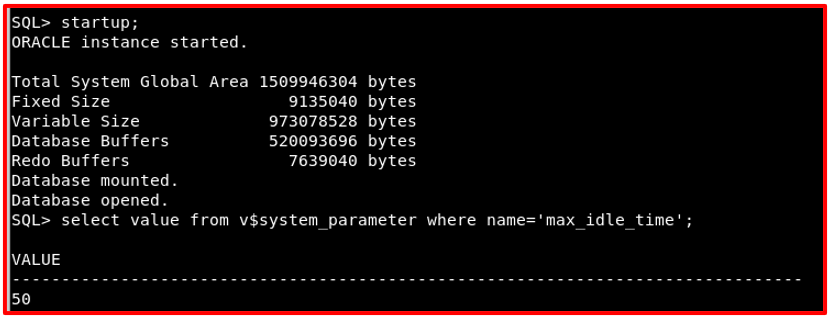Any new sessions inherited will take the value max_idle_time as 50. Now, we got a clear idea what is the difference between scope= memory and scope=spfile. When scope=memory the changes will affect the memory level. Once the database is restarted the value will be back to the original. When the scope=spfile the changes will be in spfile but we must restart the database to get the changes to the database.

##### BOTH:

Until now, we have learnt about scope=memory and scope=spfile. Now we will learn about scope=both.

Step1: Current value of the parameter max_idle_time. Let us check the current value of parameter max_idle_time.

Query: select value from v\$system_parameter where name=’max_idle_time’;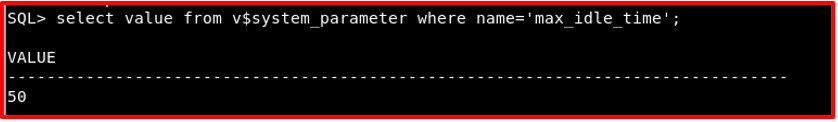Step2: If we try to change the value of the parameter you will be getting an error. So, we have to change the value of the parameter below the current value. Let us change the value from 50 to 25.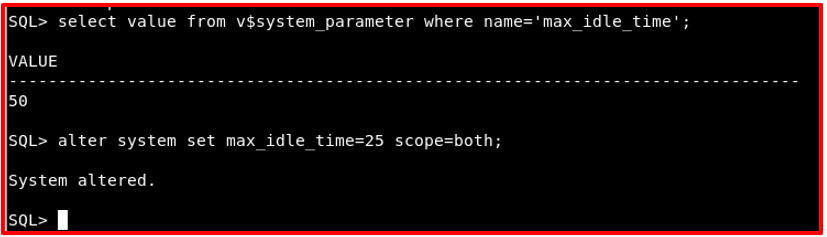So, the value of the parameter is changed to 25. Let us go ahead and the value in spfile.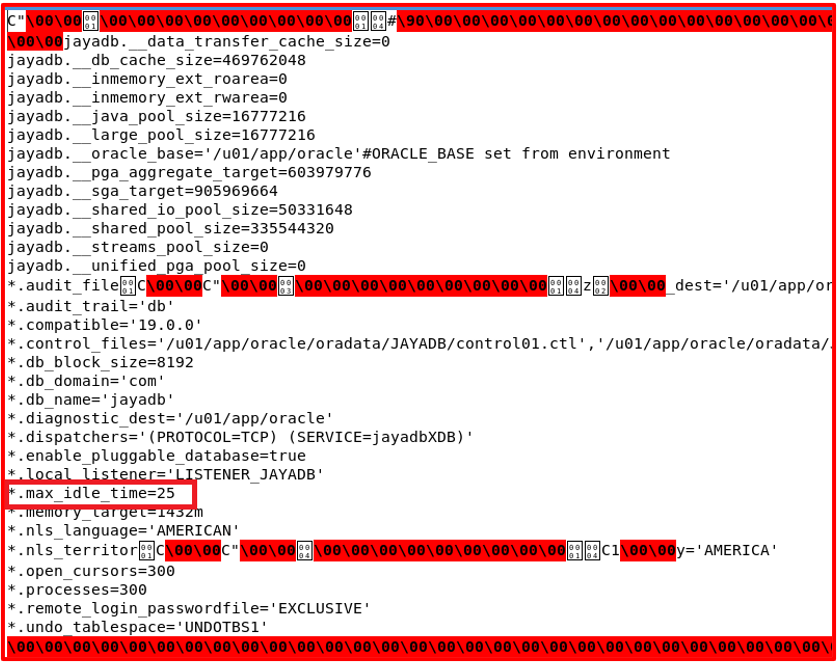We can see the value of max_idle_time is changed to 25. So, the changes are affected immediately.

Step3: Changing the value to a higher value than the current value.

Let us learn that if we try to change the value of max_idle_time from 25 to 40 we will be getting an error as below.

ERROR:
ORA-32017: failure in updating SPFILE.
ORA-02097: parameter cannot be modified because the specified value is invalid.

To solve this issue, we must reset the value of the parameter.

###### Step4: Resetting the value of any parameter.

We will try to reset the parameter max_idle_time using the below command.

Command: alter system reset max_idle_time scope=both;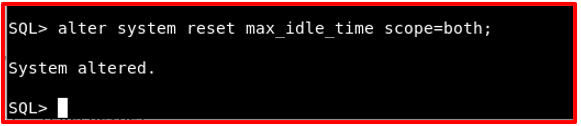Now, let us go to spfile and check the value of max_idle_time. We do not find the value of max_idle_time.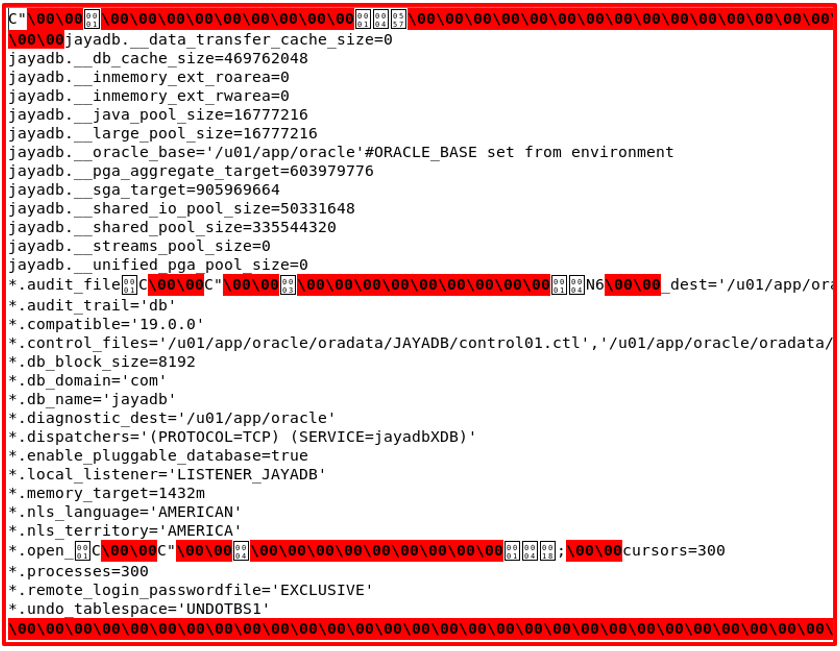From the above spfile we can see that we don’t find any value for max_idle_time.

###### Step5: Changing the max_idle_time to 100.

Let us change the value of max_idle_time to 100.

Command: alter system set max_idle_time=100 scope=both;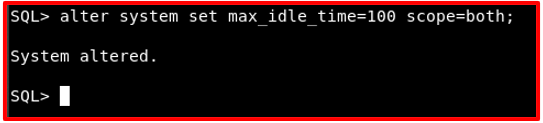Now, let us go ahead and check the value in the spfile.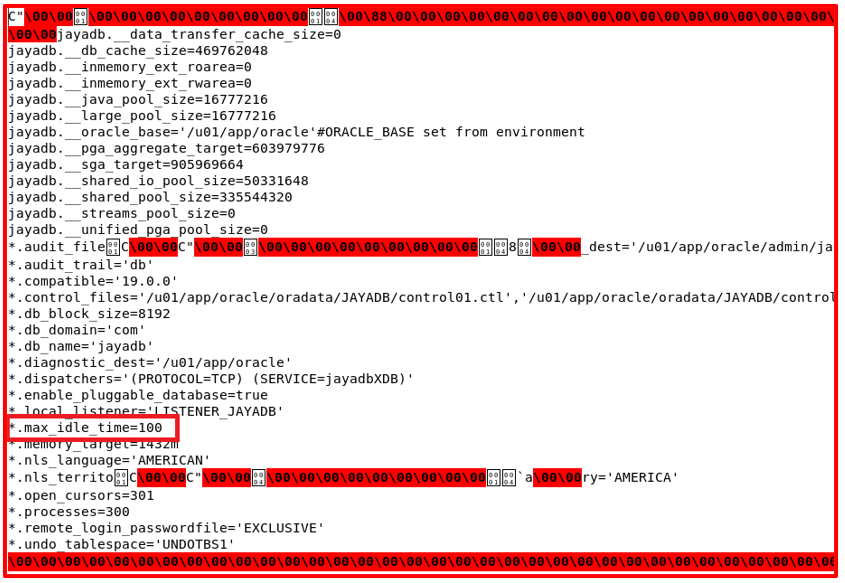We can see the value of max_idle_time=100 in spfile.

Note: Not all the parameters can be changed by scope=both. Some of the parameters will be changed using spfile and need to restart the database again to make use of those changes.

We have learnt about alter system examples where scope=memory, spfile, and both. We learn how to reset the parameter which means removing it from the spfile.

In the next article, I am going to discuss Oracle Default Scope in Alter Statement with Examples. Here, in this article, I try to explain Alter System Examples in Oracle and I hope you enjoy this Alter System Examples in Oracle article.